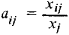# Coefficients of Direct Expenditures

## Coefficients of Direct Expenditures

(coefficients of direct expenditures for the objects of labor), sectorial indexes of expenditures of raw and processed materials, fuel, and energy to produce a unit of output.

Coefficients of direct expenditures characterize the intersectorial production links that have arisen in the national economy during the reproduction of social product. A distinction is made between report and plan coefficients of direct expenditures. Report coefficients are computed on the basis of the reported intersectorial balance of production and distribution of output and represent, in fact, specific (average) expenditures of certain products to produce a unit of another product. Plan coefficients are normative expenditures of certain products to produce a unit of another product. The development of plan coefficients creates the normative base for planning expenditures for production and is one of the most important steps in drawing up plan intersectorial balances.

Coefficients of direct expenditures are computed in physical and cost terms. In physical terms they show the material expenditure of one product needed to produce a unit of another, for example, the expenditure of coal in kilograms per 1,000 kilowatt-hours of electric power. In cost terms they show expenditures of the output of one sector to produce a unit of output of another sector in rubles.

Coefficients of direct expenditures can be expressed in the general formwhere aij is the coefficient of direct expenditures of product i to produce a unit of product j xij is the total volume of expenditures of product i to produce product j, and Xj is the total volume of product j produced. Coefficients of direct expenditures change as a result of technical progress and of improvement of the organization of production; thus, they reflect growth in the efficiency of social production.

M. R. EIDEL’MAN

Site: Follow: Share:
Open / Close